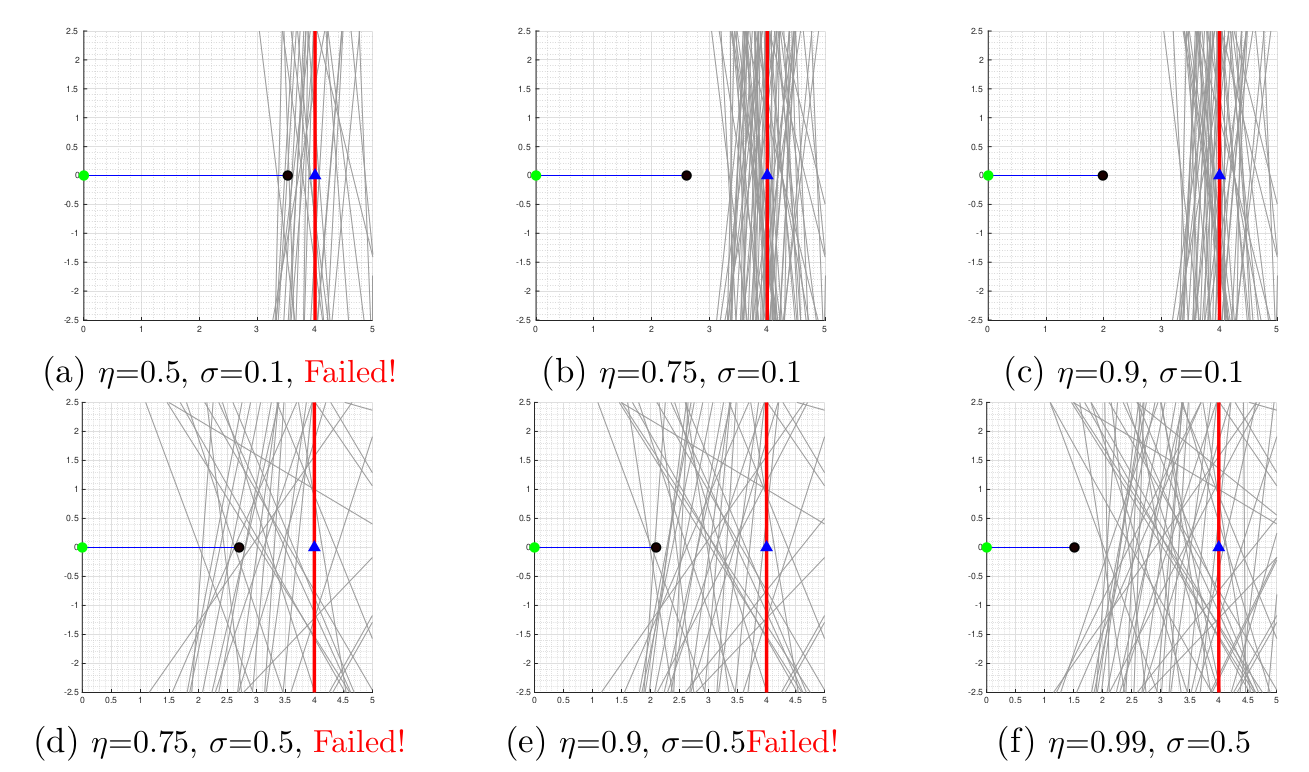# Safety against uncertain obstacles using probabilistic control barrier function

Chinmay Maheshwari, Jichan Chung, Prasanth Kotaru. EE227BT - Convex Optimization, Fall 2019. Presentation pdf

A given dynamical system is said to be safe, if the system states belong to a safe-set, i.e., $$x\in \mathcal{C}, \forall t\geq 0$$ . The safety set C is defined as a super-level set of a differential function $$h(x) : \mathbb{R}^n\rightarrow \mathbb{R},\quad \mathcal{C}=\{x: h(x)\geq 0\}$$. Typically, $$h(x)$$ is a function of system state $$x$$ and the environment, for instance, $$h(x) = dist(x, x_{obstacle} )−\varepsilon$$.

The objective is to ensure that the state $$x$$ belongs to the safety-set with high-probablity. This is achieved using the following optimization formulation,

\begin{align} \min_{(u,\delta)\in\mathcal{U}\times\mathbb{R}} & \quad u^\top u + \delta^2 \label{eq:prcbf} \\ \text{subject to} & \quad L_f V(x) + L_g V(x) u + \gamma V(x) - \delta \leq 0, \\ & \quad Pr(L_fh(x) + L_gh(x)u + \lambda h(x) \geq 0)\geq \eta \quad \text{(PrCBF)} \end{align}

For a linear barrier function, $$h = a^Tx + b$$, where $$a\sim \mathcal{N}(\bar{a}, \Sigma)$$ is a system independent normally distributed parameter. PrCBF for this system is computed as follows.

\begin{align*} &\dot{h}+\lambda h \geq 0,\\ &\nabla h^\top \dot{x} +\lambda h \geq 0,\\ &a^\top(f(x)+g(x)u)+\lambda (a^\top x+ b) \geq 0,\\ &\underbrace{a^\top f(x)}_{L_fh} +\underbrace{ a^\top g(x)}_{L_gh}u + \lambda(a^\top x+ b)\geq 0,\\ &a^\top \underbrace{ \big(- (f(x) + g(x)u +\lambda x) \big)}_{y} \leq \underbrace{\lambda b}_{\tilde{b}}. \end{align*}

For this particular problem, PrCBF is linear in the uncertain parameter $$a$$. Therefore,

$\begin{gather*} a^\top x-b\sim \mathcal{N}(\bar{a}^\top x-b,x^\top \Sigma x)\\ \textbf{Prob}(a^\top x\leq b) = \Phi\Bigg( \frac{b-\bar{a}^\top x}{\sqrt{x^\top \Sigma x}}\Bigg)\\ \textbf{Prob}(a^\top x\leq b)\geq \eta \iff b-\bar{a}^\top x\geq \Phi^{-1}(\eta)\|\Sigma^{1/2}x\|_2\\ b-\bar{a}^\top y \geq \Phi^{-1}(\eta)\|\Sigma^{1/2}y\|_2 \label{eq:socp0} \end{gather*}$

where $$y=- (f(x) + g(x)u +\lambda x)$$. The original optimization problem is thus reformulated as,

\begin{align} \min&\quad u^Tu + \delta^2\\ \text{s.t.}& L_f V(x) + L_g V(x) u + \gamma V(x) - \delta \leq 0\\ & b-\bar{a}^\top y \geq \Phi^{-1}(\eta)\|\Sigma^{1/2}y\|_2 \end{align}

### Linear System with Linear Barrier Chance Constraint

Consider a linear system $$\dot{x} = Ax +Bu$$. The optimization formulation for this system (CLF-PrCBF-SOCP) as,

$\begin{gather} \begin{matrix} {\min}_{y,\delta} & (y_0-y)^\top (y_0-y)+ {\delta^2}\\\text{s.t}& -2x^\top Py-\delta \leq -(2x^\top Py_0+ 2 x^\top PAx + \gamma x^\top Px )\\ &\tilde{b}{-}\bar{a}^\top y\geq \Phi^{-1}(\eta)\|\Sigma^{1/2}y\|_2. \end{matrix} \end{gather}$

where $$y=\underbrace{-(A+\lambda I)x}_{y_0}-Bu$$, $$\tilde{b}=\lambda b$$.

### Toy Example

Linear system with linear barrier chance constraint

$\begin{gather} A = \begin{bmatrix}0 & 0 \\ 0 & 0 \end{bmatrix}, B = \begin{bmatrix}1 & 0 \\ 0 & 1 \end{bmatrix}, h = a^Tx + b, a\in \mathcal{N}\Bigg(\begin{bmatrix} -1\\ 0\end{bmatrix}, \begin{bmatrix} \Sigma & 0 \\ 0 & \Sigma \end{bmatrix} \Bigg) \end{gather}$Simulations for various $$\eta$$ and $$\Sigma$$, the CLF-PrCBF formulation is run for various probabilities $$η \in \{0.5, 0.75, 0.9, 0.99\}$$ and different $$\Sigma \in \{0.1, 0.5\}$$. As shown in the Figure, the results are expected. Increasing $$\eta$$ ensures that the probability for safety is higher and thus the system stabilizes farther from the barrier (more conservative solution). Similarly for higher $$\Sigma$$ the system stabilizes farther from the barrier.

### References

1. Ames, Aaron D., Samuel Coogan, Magnus Egerstedt, Gennaro Notomista, Koushil Sreenath, and Paulo Tabuada. “Control barrier functions: Theory and applications.” In 2019 18th European control conference (ECC), pp. 3420-3431. IEEE, 2019.